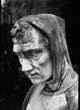﻿ DIOPHANTDIOPHANT c.214 - c.280 Greek Mathematician Diophantus of Alexandria, often known as the 'father of algebra', developed mathematical formulas for the calculation of equations and he wrote a textbook on arithmetic. From the 13 books of his main work 'Arithemtica' only six survive. 'Arithemtica' deals largely with algebra. Before Diophantus all algebra was expressed without symbolism; he was the first to introduce symbolism into Greek algebra.
 www link : Biography